Shiny从入门到入定——2-在大大的花园里面挖呀挖呀挖

# 基础 UI

## 引言

``library(shiny)``

## 输入

### 常见结构

`inputId` 有两个约束：

• 它必须是一个简单的字符串，只包含字母、数字和下划线（不允许空格、破折号、句点或其他特殊字符！）以 R 命名变量。

• 它必须是唯一的。如果它不是唯一的，那么您将无法在 server 函数中引用此控件！

``sliderInput("min", "Limit (minimum)", value = 50, min = 0, max = 100)``

### 自由文本

``````ui <- fluidPage(
textAreaInput("story", "Tell me about yourself", rows = 3)
)``````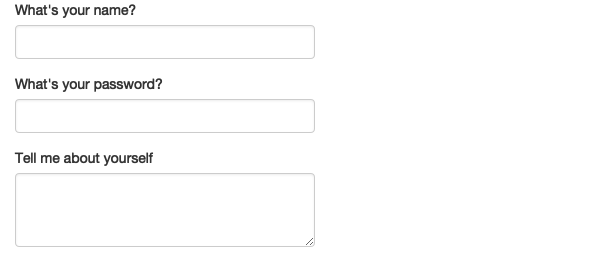### 数值输入

``````ui <- fluidPage(
numericInput("num", "Number one", value = 0, min = 0, max = 100),
sliderInput("num2", "Number two", value = 50, min = 0, max = 100),
sliderInput("rng", "Range", value = c(10, 20), min = 0, max = 100)
)``````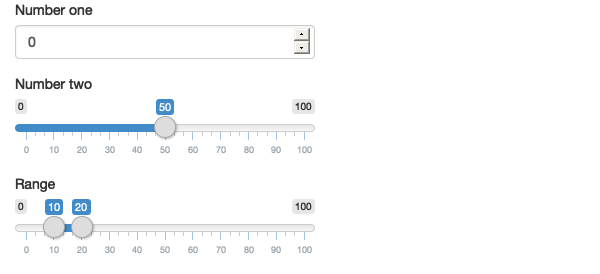### 日期

``````ui <- fluidPage(
dateInput("dob", "When were you born?"),
dateRangeInput("holiday", "When do you want to go on vacation next?")
)``````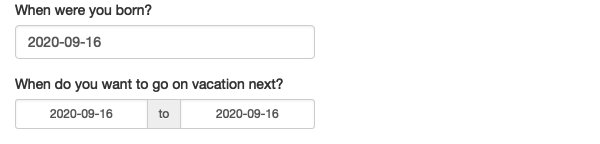### 有限制的选择

``````animals <- c("dog", "cat", "mouse", "bird", "other", "I hate animals")

ui <- fluidPage(
selectInput("state", "What's your favourite state?", state.name),
)``````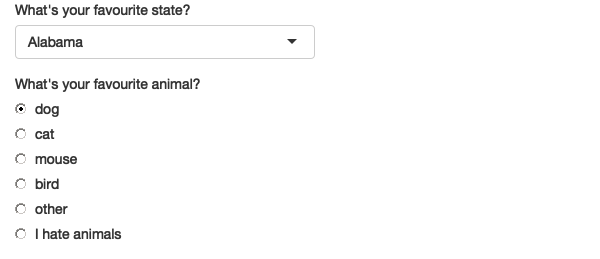``````ui <- fluidPage(
choiceNames = list(
icon("angry"),
icon("smile"),
),
)
)
#> This Font Awesome icon ('angry') does not exist:
#> * if providing a custom `html_dependency` these `name` checks can
#>   be deactivated with `verify_fa = FALSE`
#> This Font Awesome icon ('smile') does not exist:
#> * if providing a custom `html_dependency` these `name` checks can
#>   be deactivated with `verify_fa = FALSE`
#> This Font Awesome icon ('sad-tear') does not exist:
#> * if providing a custom `html_dependency` these `name` checks can
#>   be deactivated with `verify_fa = FALSE```````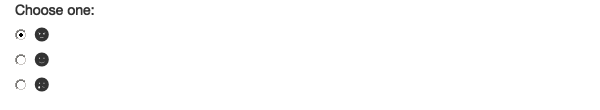``````ui <- fluidPage(
selectInput(
"state", "What's your favourite state?", state.name,
multiple = TRUE
)
)``````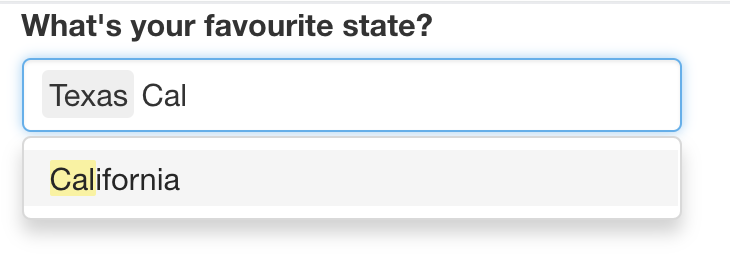``````ui <- fluidPage(
checkboxGroupInput("animal", "What animals do you like?", animals)
)``````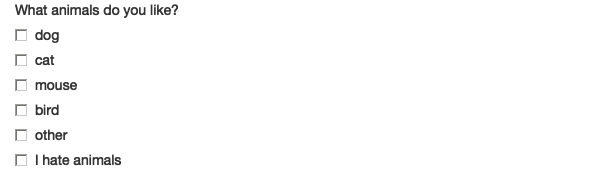``````ui <- fluidPage(
checkboxInput("cleanup", "Clean up?", value = TRUE),
checkboxInput("shutdown", "Shutdown?")
)``````

### 文件上传

``````ui <- fluidPage(
)``````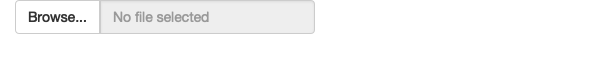fileInput () 需要在 server 端进行特殊处理，并在第 9 章中详细讨论。

### 动作按钮

``````ui <- fluidPage(
actionButton("click", "Click me!"),
actionButton("drink", "Drink me!", icon = icon("cocktail"))
)
#> This Font Awesome icon ('cocktail') does not exist:
#> * if providing a custom `html_dependency` these `name` checks can
#>   be deactivated with `verify_fa = FALSE```````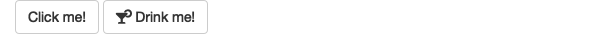``````ui <- fluidPage(
fluidRow(
actionButton("click", "Click me!", class = "btn-danger"),
actionButton("drink", "Drink me!", class = "btn-lg btn-success")
),
fluidRow(
actionButton("eat", "Eat me!", class = "btn-block")
)
)``````

`class` 参数通过设置底层 HTML 的 `class` 属性来工作，这会影响元素的样式。要查看其他选项，您可以阅读 Shiny 使用的 CSS 设计系统 Bootstrap 的文档：http://bootstrapdocs.com/v3.3.6/docs/css/#buttons

### 练习

2.2.8.1 当空间不足时，使用一个出现在文本输入区域内的占位符来标记文本框很有用。您如何调用 `textInput()` 生成下面的 UI？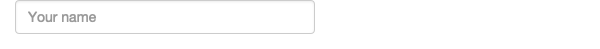2.2.8.2 认真阅读 `sliderInput()` 的文档以了解如何创建日期滑块，如下：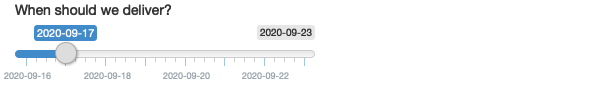2.2.8.3 如何创建日期滑块，使得用户可以在 0 到 100 之间选择日期，滑块上每个可选值的间隔为 5。然后，为输入小部件添加动画，以便当用户按下播放时，输入小部件会自动滚动到该范围。

2.2.8.4 如果您有一个中等长度的 `selectInput()` 列表，创建子标签是有用的，它可以将列表分成几部分。阅读文档以了解如何操作。（提示：底层 HTML 称为 `<optgroup>` 。）

## 输出

### 文本

``````ui <- fluidPage(
textOutput("text"),
verbatimTextOutput("code")
)
server <- function(input, output, session) {
output\$text <- renderText({
"Hello friend!"
})
output\$code <- renderPrint({
summary(1:10)
})
}``````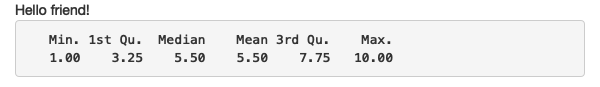``````server <- function(input, output, session) {
output\$text <- renderText("Hello friend!")
output\$code <- renderPrint(summary(1:10))
}``````

• `renderText()` 将结果合并成一个字符串，通常与 `textOutput()` 配对

• `renderPrint()` 打印结果，就像在 R 控制台中一样，通常与 `verbatimTextOutput()` 配对。

``````ui <- fluidPage(
textOutput("text"),
verbatimTextOutput("print")
)
server <- function(input, output, session) {
output\$text <- renderText("hello!")
output\$print <- renderPrint("hello!")
}``````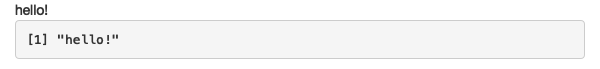### 表格

• `tableOutput()``renderTable()` 呈现静态数据表，一次显示所有数据。

• `dataTableOutput()``renderDataTable()` 呈现动态数据表，一次显示固定数量的行，同时显示控件以更改可见行。

`tableOutput()` 最适合小型、固定的摘要（例如模型系数）；如果您想向用户展示完整的数据框， `dataTableOutput()` 最为合适。如果您想更精确地控制 `dataTableOutput()` 的输出，我强烈推荐 Greg Lin 的 reactable 包。

``````ui <- fluidPage(
tableOutput("static"),
dataTableOutput("dynamic")
)
server <- function(input, output, session) {
output\$dynamic <- renderDataTable(mtcars, options = list(pageLength = 5))
}``````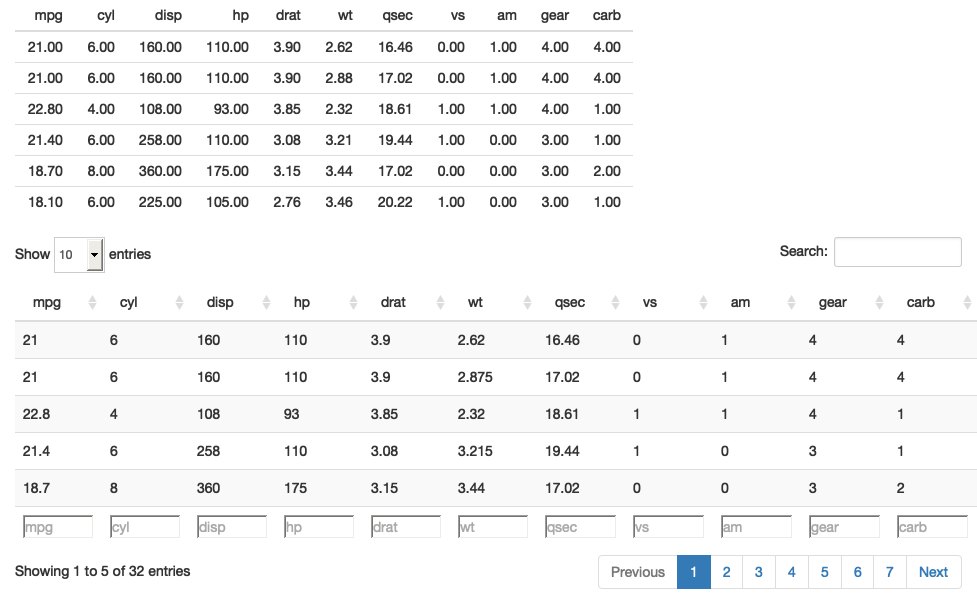### 图形

``````ui <- fluidPage(
plotOutput("plot", width = "400px")
)
server <- function(input, output, session) {
output\$plot <- renderPlot(plot(1:5), res = 96)
}``````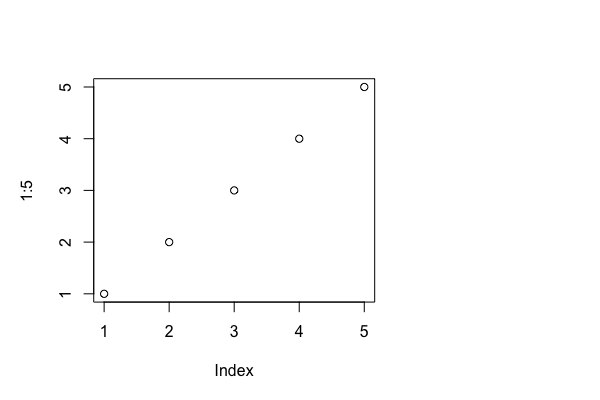### 练习

2.3.5.1 下列哪个应与 `textOutput()``verbatimTextOutput()` 配对？

a. renderPrint(summary(mtcars))

b. renderText("Good morning!")

c. renderPrint(t.test(1:5, 2:6))

d. renderText(str(lm(mpg ~ wt, data = mtcars)))

2.3.5.2 重新创建第 2.3.3 节的 Shiny 应用程序，这次将高度设置为 300 像素，宽度设置为 700 像素。设置图的 “alt” 文本，以便视障用户可以判断其是五个随机数的散点图。

2.3.5.3 更新以下调用 `renderDataTable()` 的选项，以便仅显示数据，但其他控件均被抑制（即删除搜索、排序和过滤命令）。您需要阅读？renderDataTable 并回顾 https://datatables.net/reference/option/ 中的选项。

``````ui <- fluidPage(
dataTableOutput("table")
)
server <- function(input, output, session) {
output\$table <- renderDataTable(mtcars, options = list(pageLength = 5))
}``````

2.3.5.4 或者，您可以阅读 reactable 的文档，并将上面的应用程序转换为使用它。

# 练习题答案获取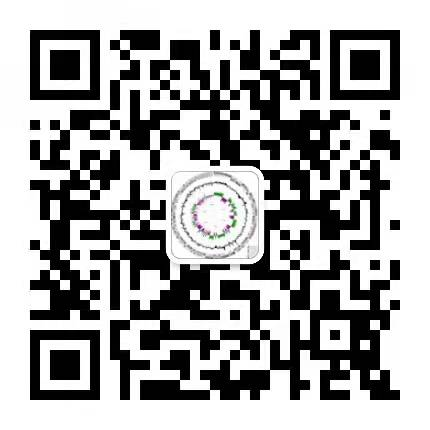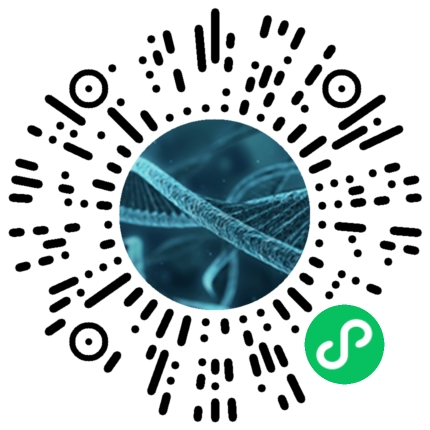16S扩增子测序及宏基因组测序